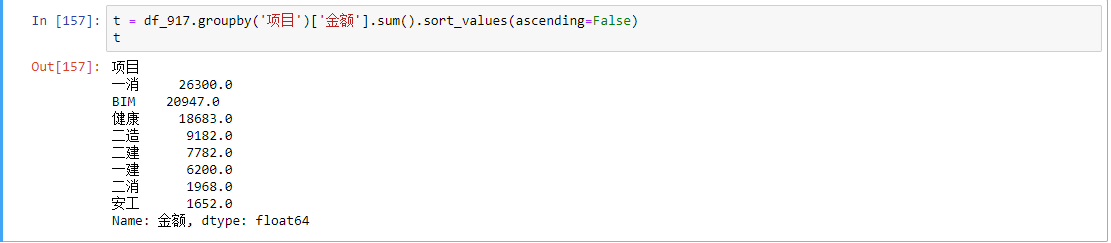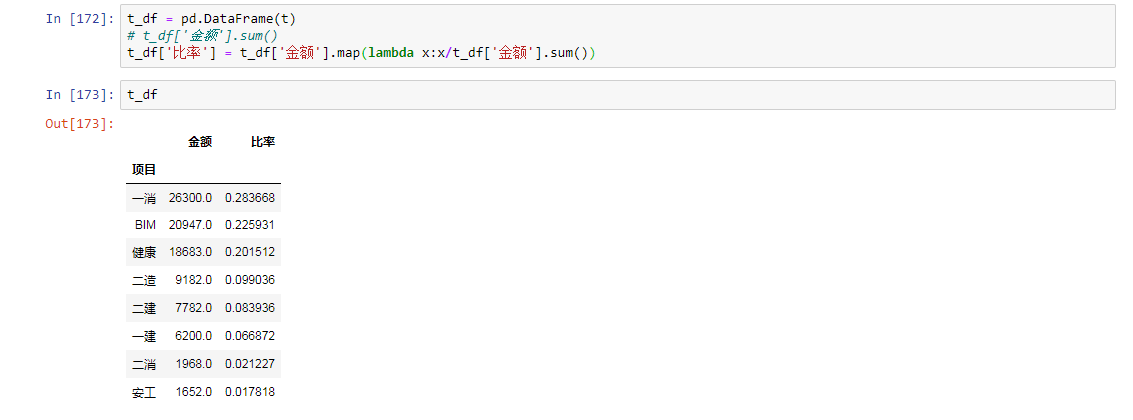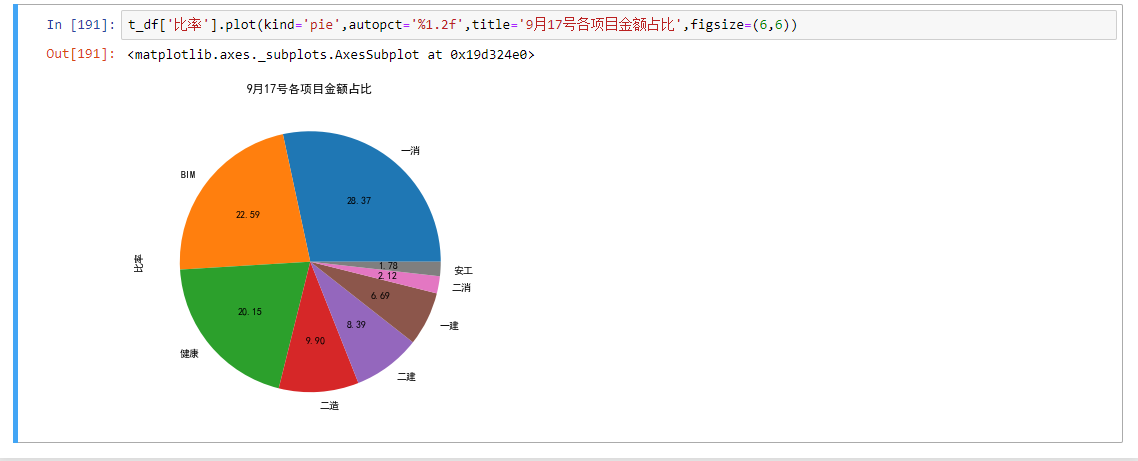• 主要为大家详细介绍了python使用matplotlib画饼状图，具有一定的参考价值，感兴趣的小伙伴们可以参考一下
• 本文实例为大家分享了python使用matplotlib画饼状图的具体代码，供大家参考，具体内容如下代码与详细注释from matplotlib import pyplot as plt#调节图形大小，宽，高plt.figure(figsize=(6,9))#定义饼状的标签，...
本文实例为大家分享了python使用matplotlib画饼状图的具体代码，供大家参考，具体内容如下
代码与详细注释
from matplotlib import pyplot as plt
#调节图形大小，宽，高
plt.figure(figsize=(6,9))
#定义饼状图的标签，标签是列表
labels = [u'第一部分',u'第二部分',u'第三部分']
#每个标签占多大，会自动去算百分比
sizes = [60,30,10]
colors = ['red','yellowgreen','lightskyblue']
#将某部分爆炸出来， 使用括号，将第一块分割出来，数值的大小是分割出来的与其他两块的间隙
explode = (0.05,0,0)
patches,l_text,p_text = plt.pie(sizes,explode=explode,labels=labels,colors=colors,
labeldistance = 1.1,autopct = '%3.1f%%',shadow = False,
startangle = 90,pctdistance = 0.6)
#labeldistance，文本的位置离远点有多远，1.1指1.1倍半径的位置
#autopct，圆里面的文本格式，%3.1f%%表示小数有三位，整数有一位的浮点数
#startangle，起始角度，0，表示从0开始逆时针转，为第一块。一般选择从90度开始比较好看
#pctdistance，百分比的text离圆心的距离
#patches, l_texts, p_texts，为了得到饼图的返回值，p_texts饼图内部文本的，l_texts饼图外label的文本
#改变文本的大小
#方法是把每一个text遍历。调用set_size方法设置它的属性
for t in l_text:
t.set_size=(30)
for t in p_text:
t.set_size=(20)
# 设置x，y轴刻度一致，这样饼图才能是圆的
plt.axis('equal')
plt.legend()
plt.show()
结果以上就是本文的全部内容，希望对大家的学习有所帮助，也希望大家多多支持我们。
本文标题: python使用matplotlib画饼状图
本文地址: http://www.cppcns.com/jiaoben/python/240234.html
展开全文• - autopct='%1.2f' 代表的是显示百分比, 显示到小数点后两位autopct='%1.2f' 代表的是显示百分比, 显示到小数点后两位
展开全文数据分析 python
• 本文实例为大家分享了python使用matplotlib画饼状图的具体代码，供大家参考，具体内容如下代码与详细注释from matplotlib import pyplot as plt#调节图形大小，宽，高plt.figure(figsize=(6,9))#定义饼状的标签，...
本文实例为大家分享了python使用matplotlib画饼状图的具体代码，供大家参考，具体内容如下
代码与详细注释
from matplotlib import pyplot as plt
#调节图形大小，宽，高
plt.figure(figsize=(6,9))
#定义饼状图的标签，标签是列表
labels = [u'第一部分',u'第二部分',u'第三部分']
#每个标签占多大，会自动去算百分比
sizes = [60,30,10]
colors = ['red','yellowgreen','lightskyblue']
#将某部分爆炸出来， 使用括号，将第一块分割出来，数值的大小是分割出来的与其他两块的间隙
explode = (0.05,0,0)
patches,l_text,p_text = plt.pie(sizes,explode=explode,labels=labels,colors=colors,
labeldistance = 1.1,autopct = '%3.1f%%',shadow = False,
startangle = 90,pctdistance = 0.6)
#labeldistance，文本的位置离远点有多远，1.1指1.1倍半径的位置
#autopct，圆里面的文本格式，%3.1f%%表示小数有三位，整数有一位的浮点数
#startangle，起始角度，0，表示从0开始逆时针转，为第一块。一般选择从90度开始比较好看
#pctdistance，百分比的text离圆心的距离
#patches, l_texts, p_texts，为了得到饼图的返回值，p_texts饼图内部文本的，l_texts饼图外label的文本
#改变文本的大小
#方法是把每一个text遍历。调用set_size方法设置它的属性
for t in l_text:
t.set_size=(30)
for t in p_text:
t.set_size=(20)
# 设置x，y轴刻度一致，这样饼图才能是圆的
plt.axis('equal')
plt.legend()
plt.show()
结果以上就是本文的全部内容，希望对大家的学习有所帮助，也希望大家多多支持脚本之家。
展开全文• from matplotlib import pyplot as plt #调节图形大小，宽，高 plt.figure(figsize=(6,9)) #定义饼状的标签，标签是列表 labels = [u'第一部分',u'第二部分',u'第三部分'] #每个标签占多大，会自动去算百分比 ...
 代码与详细注释
from matplotlib import pyplot as plt

#调节图形大小，宽，高
plt.figure(figsize=(6,9))
#定义饼状图的标签，标签是列表
labels = [u'第一部分',u'第二部分',u'第三部分']
#每个标签占多大，会自动去算百分比
sizes = [60,30,10]
colors = ['red','yellowgreen','lightskyblue']
#将某部分爆炸出来， 使用括号，将第一块分割出来，数值的大小是分割出来的与其他两块的间隙
explode = (0.05,0,0)

patches,l_text,p_text = plt.pie(sizes,explode=explode,labels=labels,colors=colors,
labeldistance = 1.1,autopct = '%3.1f%%',shadow = False,
startangle = 90,pctdistance = 0.6)

#labeldistance，文本的位置离远点有多远，1.1指1.1倍半径的位置
#autopct，圆里面的文本格式，%3.1f%%表示小数有三位，整数有一位的浮点数
#startangle，起始角度，0，表示从0开始逆时针转，为第一块。一般选择从90度开始比较好看
#pctdistance，百分比的text离圆心的距离
#patches, l_texts, p_texts，为了得到饼图的返回值，p_texts饼图内部文本的，l_texts饼图外label的文本

#改变文本的大小
#方法是把每一个text遍历。调用set_size方法设置它的属性
for t in l_text:
t.set_size=(30)
for t in p_text:
t.set_size=(20)
# 设置x，y轴刻度一致，这样饼图才能是圆的
plt.axis('equal')
plt.legend()
plt.show()
结果饼状图


展开全文python
• matplotlib.pyplot.pie(x, explode=None, labels=None, colors=None, autopct=None, pctdistance=0.6, shadow=False, labeldistance=1.1, startangle=None, radius=None, counterclock=True, wedgeprops=None, ...
• import matplotlib.pyplot as plt plt.figure(figsize=(6,6)) # 将画布设定为正方形 label='A','B','C','D','E' # 各类别标签 sizes=[35,25,18,12,10] # 各类别占比 color='g','r','b','y','c' # 各类别颜色 explode...python
• from matplotlib import pyplot as plt #调节图形大小，宽，高 plt.figure(figsize=(6,9)) #定义饼状的标签，标签是列表 labels = [u'第一部分',u'第二部分',u'第三部分'] #每个标签占多大，会自动去算百分比 ...
• 遇到一个问题，就是画饼状图的时候，存在数据堆叠的情况。 废话少说，首先看个例子： 但是我想要的是这种情况： 于是我就找方法，找到了一种方法是生成html文件格式的情况：参考...
• from matplotlib import pyplot as plt #调节图形大小，宽，高 plt.figure(figsize=(6,9)) #定义饼状的标签，标签是列表 labels = [u'第一部分',u'第二部分',u'第三部分'] #每个标签占多大，会自动去算百分比 ...
• from matplotlib import pyplot as plt #调节图形大小，宽，高 plt.figure(figsize=(6,9)) #定义饼状的标签，标签是列表 labels = [u'第一部分',u'第二部分',u'第三部分'] #每个标签占多大，会自动去算百分比 ...python
• from matplotlib import pyplot as plt#调节图形大小，宽，高plt.figure(figsize=(6,9))#定义饼状的标签，标签是列表labels = [u'第一部分',u'第二部分',u'第三部分']#每个标签占多大，会自动去算百分比sizes = ...
• 主要介绍了python使用Matplotlib画饼图，具有一定的参考价值，感兴趣的小伙伴们可以参考一下
• 用Python的matplotlib画饼图： 代码： #饼图：超市主要商品本月销售量 import matplotlib.pyplot as plt plt.title('Sales of major commodities in supermarkets for this month') labels = 'Noodles', 'Milk', '...
• 画饼画使用的方法是plt.pie方法，里面有几个参数可以设置，具体后面再提，先看下代码和效果。这里以常见的几个课目的值为例，算下各个科目在饼图中占的比重。import matplotlib.pyplot as pltx = [4, 9, 21, 55, 30,...
• 画饼画使用的方法是plt.pie方法，里面有几个参数可以设置，具体后面再提，先看下代码和效果。这里以常见的几个课目的值为例，算下各个科目在饼图中占的比重。import matplotlib.pyplot as pltx = [4, 9, 21, 55, 30,...
• 我写的这个函数是画饼的，有两个参数，一个是字典，字典的内容例如’a’=7，就是前面是字符串后面是数字，运行完后会在当前路径下输入运行的结果。 def Plo2class(Dir,title): New_Dir=Dir.copy() Null_List...
• ## Python中使用matplotlib画饼图详解

万次阅读 多人点赞 2017-05-29 00:10:27
本文来自本人做的一个案例，经过比较，个人感觉Python中的matplotlib要比excel、R语言中ggplot2中的饼图好看。 解决画图中的中文乱码问题 参数详解 保存结果 解决画图中的中文乱码问题 为防止中文乱码问题，在...python ggplot2
• 本文实例为大家分享了Android九宫格图片展示的具体代码，供大家参考，具体内容如下函数参数plt.pie(x, explode=None, labels=None, colors=None,autopct=None, pctdistance=0.6, shadow=False,labeldistance=1.1, ...
• https://blog.csdn.net/u012111465/article/details/72797032本文来自本人做的一个案例，经过比较，个人感觉Python中的matplotlib要比excel、R语言中ggplot2中的饼图好看。解决画图中的中文乱码问题参数详解保存......